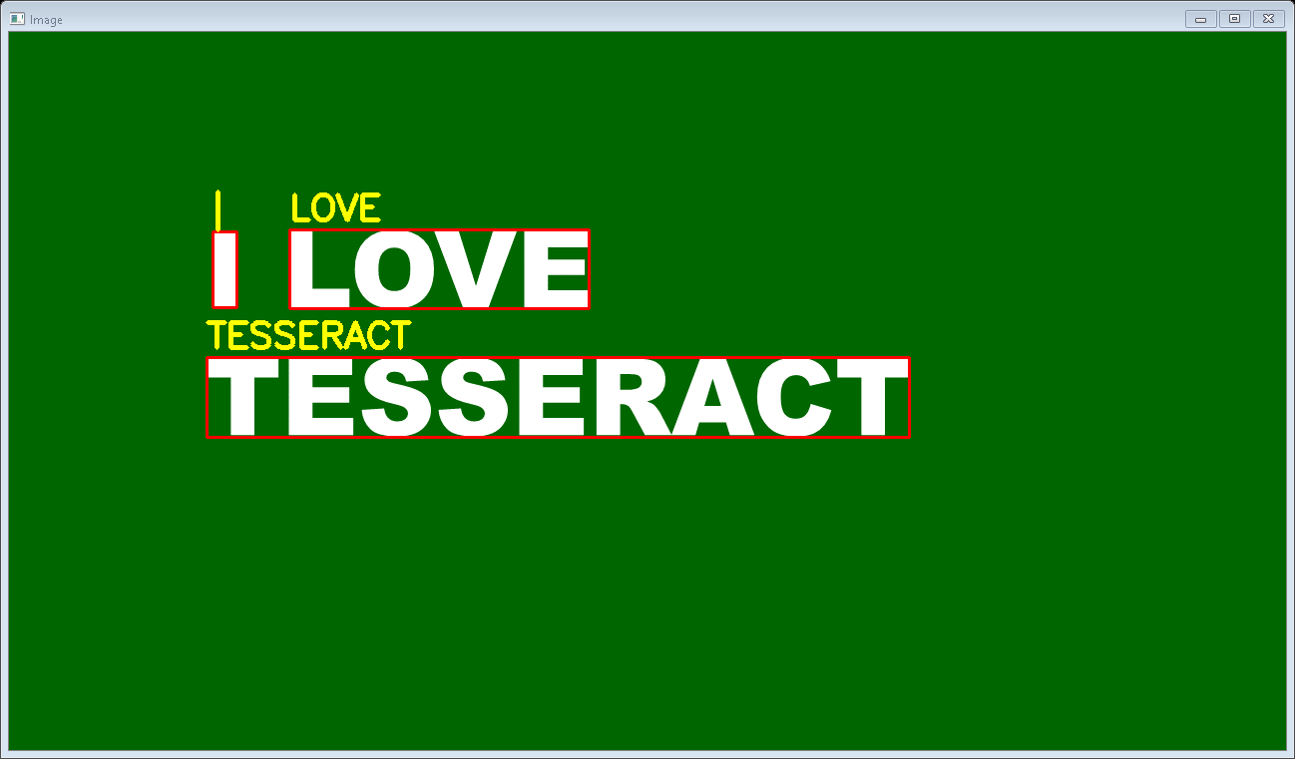# Text Localization, Detection and Recognition using Pytesseract

Pytesseract or Python-tesseract is an Optical Character Recognition (OCR) tool for Python. It will read and recognize the text in images, license plates etc. Python-tesseract is actually a wrapper class or a package for Google’s Tesseract-OCR Engine. It is also useful and regarded as a stand-alone invocation script to tesseract, as it can easily read all image types supported by the Pillow and Leptonica imaging libraries, which mainly includes –

• jpg
• png
• gif
• bmp
• tiff etc

Also additionally, if it is used as a script, Python-tesseract will also print the recognized text instead of writing it to a file. Python-tesseract can be installed using pip as shown below –

```pip install pytesseract
```

If you are using Anaconda Cloud, Python-tesseract can be installed as shown below:-

```conda install -c conda-forge/label/cf202003 pytesseract
```

or

```conda install -c conda-forge pytesseract
```

Note: tesseract should be installed in the system before running the below script.

Below is the implementation.

 `from` `pytesseract ``import``*` `import` `argparse ` `import` `cv2 ` ` `  ` `  `# We construct the argument parser ` `# and parse the arguments ` `ap ``=` `argparse.ArgumentParser() ` ` `  `ap.add_argument(``"-i"``, ``"--image"``, ` `                ``required``=``True``, ` `                ``help``=``"path to input image to be OCR'd"``) ` `ap.add_argument(``"-c"``, ``"--min-conf"``, ` `                ``type``=``int``, default``=``0``, ` `                ``help``=``"mininum confidence value to filter weak text detection"``) ` `args ``=` `vars``(ap.parse_args()) ` ` `  `# We load the input image and then convert ` `# it to RGB from BGR. We then use Tesseract ` `# to localize each area of text in the input ` `# image ` `images ``=` `cv2.imread(args[``"image"``]) ` `rgb ``=` `cv2.cvtColor(images, cv2.COLOR_BGR2RGB) ` `results ``=` `pytesseract.image_to_data(rgb, output_type``=``Output.``DICT``) ` ` `  `# Then loop over each of the individual text ` `# localizations ` `for` `i ``in` `range``(``0``, ``len``(results[``"text"``])): ` `     `  `    ``# We can then extract the bounding box coordinates ` `    ``# of the text region from  the current result ` `    ``x ``=` `results[``"left"``][i] ` `    ``y ``=` `results[``"top"``][i] ` `    ``w ``=` `results[``"width"``][i] ` `    ``h ``=` `results[``"height"``][i] ` `     `  `    ``# We will also extract the OCR text itself along ` `    ``# with the confidence of the text localization ` `    ``text ``=` `results[``"text"``][i] ` `    ``conf ``=` `int``(results[``"conf"``][i]) ` `     `  `    ``# filter out weak confidence text localizations ` `    ``if` `conf > args[``"min_conf"``]: ` `         `  `        ``# We will display the confidence and text to ` `        ``# our terminal ` `        ``print``(``"Confidence: {}"``.``format``(conf)) ` `        ``print``(``"Text: {}"``.``format``(text)) ` `        ``print``("") ` `         `  `        ``# We then strip out non-ASCII text so we can ` `        ``# draw the text on the image We will be using ` `        ``# OpenCV, then draw a bounding box around the ` `        ``# text along with the text itself ` `        ``text ``=` `"".join(text).strip() ` `        ``cv2.rectangle(images, ` `                      ``(x, y), ` `                      ``(x ``+` `w, y ``+` `h), ` `                      ``(``0``, ``0``, ``255``), ``2``) ` `        ``cv2.putText(images, ` `                    ``text, ` `                    ``(x, y ``-` `10``),  ` `                    ``cv2.FONT_HERSHEY_SIMPLEX, ` `                    ``1.2``, (``0``, ``255``, ``255``), ``3``) ` `         `  `# After all, we will show the output image ` `cv2.imshow(``"Image"``, images) ` `cv2.waitKey(``0``) `

Output:
Execute the command below to view the Output

```python ocr.py --image ocr.png
```In addition to Output, we will see the Confidence Level and the Text In Command Prompt as shown below –

```Confidence: 93
Text: I

Confidence: 93
Text: LOVE

Confidence: 91
Text: TESSERACT
```

My Personal Notes arrow_drop_upCheck out this Author's contributed articles.

If you like GeeksforGeeks and would like to contribute, you can also write an article using contribute.geeksforgeeks.org or mail your article to contribute@geeksforgeeks.org. See your article appearing on the GeeksforGeeks main page and help other Geeks.

Please Improve this article if you find anything incorrect by clicking on the "Improve Article" button below.

Improved By : Akanksha_Rai

Article Tags :

1

Please write to us at contribute@geeksforgeeks.org to report any issue with the above content.